Precalculus by Richard Wright

Are you not my student and
has this helped you?The Lord is my rock, my fortress and my deliverer; my God is my rock, in whom I take refuge, my shield and the horn of my salvation, my stronghold. Psalms‬ ‭18‬:‭2‬ ‭NIV‬‬‬‬‬‬

# 3-05 Exponential and Logarithmic Models

Summary: In this section, you will:

• Use exponential growth and decay models.
• Use the Gaussian model.
• Use the logistic growth model.
• Use logarithmic models.

Exponential models are often used to model population. Consider rabbits. They breed quickly and a rabbit population can quickly grow. If each rabbit has six babies, this would be exponential growth with the base of 6. The population will not grow exponentially forever, some rabbits will die from old age, predators, or starvation. As the number of rabbits increase, the available food supply is does not change, so their will not be enough food for all the rabbits and some will starve. The population will eventually level out and quit growing. This population level is called the carrying capacity. In this lesson, various exponential and logarithmic models will be discussed and used to solve real world problems.

## Exponential Growth and Decay Models

Exponential growth occurs when the value of something is repeatedly multiplied by the same number greater than 1. Exponential decay is the same idea only multiplied by a number between 0 and 1. Repeated multiplication is exponents, so the model is

y = A0ekt

where A0 is the original quantity, k is the growth constant, and t is time. Figure 2a shows exponential growth and Figure 2b shows exponential decay.

###### Exponential Growth and Decay

y = A0ekt

where y = current amount
A0 = initial amount
k = decay constant (if k > 0 it is growth; if k < 0 it is decay)
t = time

#### Example 1: Exponential Growth

A population of bacteria doubles every hour. If the culture started with 10 bacteria, graph the population as a function of time.

###### Solution

This is exponential growth so the model is

y = A0ekt

The initial population was 10, so A0 = 10. The population doubles every hour, so at t = 1, y = 20. Plug this information into the model and find k.

y = A0ekt
20 = 10ek1
2 = ek
ln 2 = ln ek
ln 2 = k

Fill in k to the model and simplify.

y = 10e(ln 2)t
y = 10(eln 2)t
y = 10 ⋅ 2t

Now graph like was done in Lesson 3-01. The asymptote is y = 0, domain is [0, ∞) because we cannot go backward in time, and the range is [10, ∞) because 10 is the initial quantity. Now make a table of values and graph.

##### Try It 1

The number of candies in a candy dish are eaten in such a way that a third of them disappear every day because no one wants to look like they are eating all the candies. If there were initially 300 candies in the dish, how many are left after a 5-day work week?

40 candies remain

Unstable atomic isotopes of an element have a nucleus that will spontaneously change to another element, called a daughter element, releasing radiation. This decay happens at a set rate, at any given moment there is a set probability that the nucleus will decay into daughter elements. For example, Carbon-14 decays into stable Nitrogen-14. The half-life, or amount of time for half of a sample to decay, of 14C is about 5700 years.

Radioactive decay using 14C is used to date samples organic material with a limit to about 50,000 years and other radioactive elements are used to date samples of rock with years ranging from about 200,000 years to hundreds of millions of years. Many Christian creationists feel the Bible indicates the Earth was created no later than 10,000 years ago, so radiometric dating poses a potential problem. The dates are typically consistent, so that deeper down rocks are generally given older dates than rocks higher up in the earth. However, radiometric dating is more complicated than using the simple exponential decay formula. For radiometric dating, there are some assumptions that are used and adjustments are made because of these assumptions.

1. The amount of daughter elements of the original sample is known.
2. None of the radioactive element or the daughter element has been added to, or taken away from, the sample.
3. The decay rate has not changed since the sample was formed.

If the assumptions are not valid, then the calculated dates are wrong. There is some debate about the validity of each of these assumptions. See here for more information.

Radiometric and radiocarbon dating is a controversal subject. Secular scientists would like everyone to believe it is a foolproof method with no substatial problems. Carbon-14 dating does seem to work well for the historical time period where the method has been calibrated using historical artifacts. Before that is extrapolation which may or may not be accurate. Creation scientists would like to point out the shortcomings and possible issues with the methods because each person should understand both sides of the issue and encourage furthur open-minded research.

For the purposes of this lesson, we will use the simple exponential decay model from above.

A = A0ekt

The decay constant, k, can be found by knowing the half-life. The half-life is the amount of time for half of the sample to radioactively decay. For example, if an experiment starts with 4 mg of 14C and the half-life of 14C is 5700 years, then after 5700 years there will only be 2 mg of 14C left. This model is true for large samples, but not for individual atoms where probabilities come into play. To find the decay constant, replace A with ½A0 and solve for k.

The half-life of Carbon-14 is about 5700 years. If the sample currently has 1.5 mg of 14C, how much did it have 2,000 years ago?

###### Solution

First find k by plugging in A = 1.5, A = ½A0 = 1.5 so A0 = 3, and t = 5700.

A = A0ekt
1.5 = 3ek5700
0.5 = e5700k
ln 0.5 = ln e5700k
ln 0.5 = 5700k
$k=\frac{ln0.5}{5700}\approx -0.0001216$

Now plug in k ≈ −0.0001216, A = 1.5, and t = 2,000 and solve for A0.

A = A0ekt
1.5 = A0e(−0.0001216 ⋅ 2,000)
1.5 = A0(0.7841)
1.913 = A0

So, 2,000 years ago, there would have been 1.913 mg of 14C.

##### Try It 2

Potassium-40 has a half-life of 1.251×109 years. If there is initially 5 g of 40K, how much will there be after 5,000 years?

4.99999 g

## Logistic Growth Model

Exponential growth cannot continue forever. Consider the rabbits from the beginning of the lesson. Each pair of rabbits produces an average litter of 6 babies every 1 to 2 months. Eventually there will be so many rabbits that there will not be enough food for them to all live, so fewer rabbits will be born. Eventually the number of rabbits will plateau at the carrying capacity. With constants a, b, and c, the model is

$f\left(t\right)=\frac{c}{1+a{e}^{-bt}}$

###### Logistic Growth Model

$f\left(t\right)=\frac{c}{1+a{e}^{-bt}}$

where $\frac{c}{1+a}$ = initial value,
c = carrying capacity,
b = constant determined by the rate of growth

#### Example 3: Logistic Growth Model

An influenza epidemic spreads through a population rapidly, at a rate that depends on two factors: The more people who have the flu, the more rapidly it spreads, and also the more uninfected people there are, the more rapidly it spreads. These two factors make the logistic model a good one to study the spread of communicable diseases. And, clearly, there is a maximum value for the number of people infected: the entire population.

For example, at time t = 0 there is one person in a community of 1,000 people who has the flu. So, in that community, at most 1,000 people can have the flu. Researchers find that for this particular strain of the flu, the logistic growth constant is b = 0.6030. (a) Estimate the number of people in this community who will have had this flu after ten days. (b) Predict how many people in this community will have had this flu after a long period of time has passed.

###### Solution

Substitute the given data into the logistic growth model.

$f\left(t\right)=\frac{c}{1+a{e}^{-bt}}$

Because at most 1,000 people, the entire population of the community, can get the flu, we know the limiting value is c = 1000. To find a, we use the formula that the number of cases at time t = 0 is $$1 = \frac{1000}{1 + ae^{-b\left(0\right)}}$$ so, $1=\frac{1}{1000+a}$, from which it follows that a = 999.

1. This model predicts that, after ten days, the number of people who have had the flu is $f\left(t\right)=\frac{1000}{1+999{e}^{-0.6030t}}\approx 293.8$. Because the actual number must be a whole number (a person has either had the flu or not) we round to 294.
2. In the long term, the number of people who will contract the flu is the limiting value, c = 1000.

Analysis

Remember that, because we are dealing with a virus, we cannot predict with certainty the number of people infected. The model only approximates the number of people infected and will not give us exact or actual values. The graph in figure 5 gives a good picture of how this model fits the data.

##### Try It 3

Using the model in Example 3, estimate the number of cases of flu on day 15.

895

## Gaussian Model

Data sets such as SAT scores and heights of women in America fit the Gaussian Model which is often used in statistics to model the normal distribution. The Gaussian Model is called the normal distribution because the distribution many large data sets align with it. The mean value is in the center with less common values on each side. The shape to the Gaussian Model's graph is often called a bell curve.

The Gaussian Model function is

f(x) = ae−(xb)2/c

The average value of the data is the x-value corresponding to the central highest y-value.

###### Gaussian Model

f(x) = ae−(xb)2/c

where a = maximum value
b = mean (average value)
c = determines the steepness of the sides

#### Example 4: Gaussian Model

According to the National Center for Education Statistics, the national results of the 2009 ACT test roughly fits the normal distribution model

y = 0.25e−(x − 21.1)2/52.02; 1 ≤ x ≤ 36

Graph this function. Then determine the average ACT score in 2009.

###### Solution

Make a table of values to graph the function. Notice that a = 0.25, so that is the maximum y-value. The domain is given in the problem so only use x-values between 1 and 36.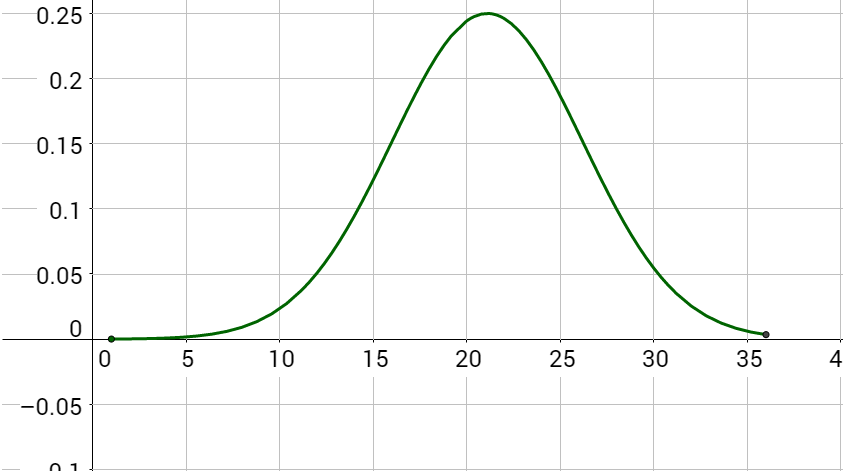Figure 7 y = 0.25e−(x − 21.1)2/52.02; 1 ≤ x ≤ 36

Notice the highest point of the graph has an x-value just a little more than 20. By comparing the function with the Gaussian model, b = 21.1 which is the average.

## Logarithmic Models

In physics, how human perceive intensity of events such as sound or earthquakes is made into a logarithmic scale. For example the Richter scale for earthquakes is

$R=log\frac{I}{{I}_{0}}$

where R is the magnitude of the earthquake, I is the intensity of the earthquake, and I0 is 1 which is the minimum possible intensity used for comparison.

#### Example 5: Richter Scale

Find the intensity of each earthquake.

1. In 2015, there was an earthquake in Galesburg, Michigan of magnitude 4.2. (The second strongest in Michigan history.) (Source: earthquaketrack.com)
2. In 1960, the strongest ever earthquake was in Valdivia, Chile with a magnitude of 9.5. (Source: USGS)
###### Solution
1. Use the Ritcher model above with R = 4.2 and I0 = 1. Then solve for I.

$R=log\frac{I}{{I}_{0}}$
$4.2=log\frac{I}{1}$
4.2 = log I
104.2 = 10log I
15,849 ≈ I

2. Use the Ritcher model above with R = 9.5 and I0 = 1. Then solve for I.

$R=log\frac{I}{{I}_{0}}$
$9.5=log\frac{I}{1}$
9.5 = log I
109.5 = 10log I
3,162,277,660 ≈ I

##### Try It 4

Find the intensity of a magnitude 5 earthquake.

100,000

##### Lesson Summary

###### Exponential Growth and Decay

y = A0ekt

where y = current amount
A0 = initial amount
k = decay constant (if k > 0 it is growth; if k < 0 it is decay)
t = time

###### Logistic Growth Model

$f\left(t\right)=\frac{c}{1+a{e}^{-bt}}$

where $\frac{c}{1+a}$ = initial value,
c = carrying capacity,
b = constant determined by the rate of growth

###### Gaussian Model

f(x) = ae−(xb)2/c

where a = maximum value
b = mean (average value)
c = determines the steepness of the sides

## Practice Exercises

1. Is half-life associated with exponential growth or decay? What about doubling time?
2. Quickly sketch a graph of
1. exponential growth
2. exponential decay
3. logarithmic
4. logistic
5. Gaussian
3. The number of people in a certain city infected by a flu virus doubles every 3 days. If the flu started with a household of 4 people, how many people have been infected after 25 days?
4. Suzy has a headache so she takes a regular 325 mg aspirin tablet. The half-life of aspirin in the blood is about 15 minutes. How much aspirin remains in her system after 2 hours?
5. In a certain crate of 250 apples, 20% rot each day. How many good apples remain after one week?
6. A common chemotherapy drug to treat cancer is 5-Fuorouracil. Each hour, 83.9% of it decays. A 75 kg patient is given a 2250 mg dose.
1. Write an equation to model the amount of 5-Fluorouracil in the body after t hours.
2. How much of the drug remains after 6 hours?
3. How long until one quarter of the drug remains?
7. Use the logistic growth model $$f(x) = \frac{200}{1 + 12e^{-0.5x}}$$.
1. Find and interpret f(0).
2. Find the carrying capacity.
8. The number of sparrows in a backyard after t years can be modeled by $$S(t) = \frac{125}{1+17e^{-0.452t}}$$.
1. Graph the function.
2. What is the initial population of sparrows?
3. How many sparrows are in the backyard after 5 years?
4. How long will it take for the population to reach 90 birds?
5. What is the carrying capacity?
9. The function $$R(t) = \frac{230}{1+45e^{-0.6t}}$$ models the number of students at a school who have heard a certain rumor after t days.
1. How many people started the rumor?
2. How many people have heard the rumor after 4 days?
3. As t increases, what value does R(t) approach? Interpret your answer.
4. Read Proverbs 14:15. What does the wise man suggest about believing what you hear?
10. The IQ scores for a sample of a class are approximated by the normal distribution $y=0.03{e}^{-\frac{{\left(x-100\right)}^{2}}{450}},70\le x\le 115$, where x is the IQ score.
1. Graph the function.
2. Find the average IQ score.
11. In a small college, the amount of time in hours per week a student uses a math-tutoring center approximates the normal distribution $f\left(t\right)=0.7979{e}^{-\frac{{\left(t-5.4\right)}^{2}}{0.5}}$, 4 ≤ t ≤ 7, where t is the number of hours.
1. Graph the function.
2. What is the average number of hours per week that a students uses the center?
12. Use the Richter scale $R=log\frac{I}{{I}_{0}}$ and let I0 = 1.
1. Find the intensity of the 2.5 magnitude earthquake in Alpena, Michigan in 2015.
2. Find the intensity of the 5.8 magnitude earthquake in Louisa, Virginia that damaged Washington, D.C. in 2011.
3. Find the magnitude, R, of an earthquake with I = 10,000,000.
4. Find the magnitude, R, of an earthquake with I = 20,000.
13. The sound level in decibels is given by the formula $\beta =10log\left(\frac{I}{{I}_{0}}\right)$ where β is the sound level, I is the sound intensity, and I0 is 10−12 W/m2 or the softest audible sound.
1. Find the sound level, β, if I = 10−7 W/m2 (average home).
2. Find the sound level, β if I = 10−1 W/m2 (chainsaw at 1 m).
3. Find the intensity, I, if β = 90 dB (diesel truck at 10 m).
4. Find the intenstiy, I, if β = 10 dB (rustling leaves).
14. Enter the data from each table into a graphing calculator and graph the resulting scatter plots. Determine whether the data from the table could represent a function that is linear, exponential, or logarithmic.

15.  x f(x) 1 2 3 4 5 6 7 8 9 10 -0.631 0 0.369 0.631 0.834 1 1.14 1.262 1.369 1.465
16.  x f(x) 1 2 3 4 5 6 7 8 9 10 1.2 1.44 1.728 2.074 2.488 2.986 3.583 4.3 5.16 6.192
17. Mixed Review

18. (3-04) Solve 2 + 3 log3 (x + 1) = 4.
19. (3-04) Solve 5 ⋅ 4t + 3 = 10.
20. (3-03) Expand ${log}_{5}\left(\frac{5r}{{t}^{3}}\right)$.
21. (3-02) Solve by rewriting as an exponential equation: 5 = log2 x.
22. (3-01) The graph of f(x) = 5x is reflected over the x-axis and stretched vertically by a factor of 3. Then it is shifted 2 units left and 4 units up. What is the equation of the new function, g(x)? State its y-intercept, domain, and range.

1. Exponential decay; Exponential growth
2.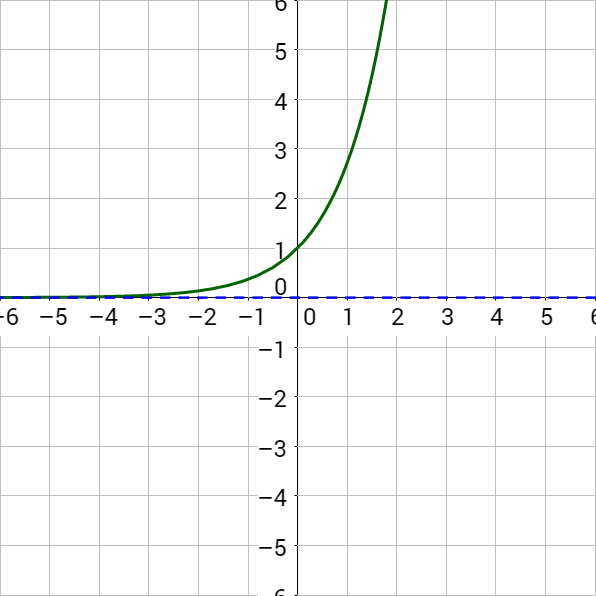;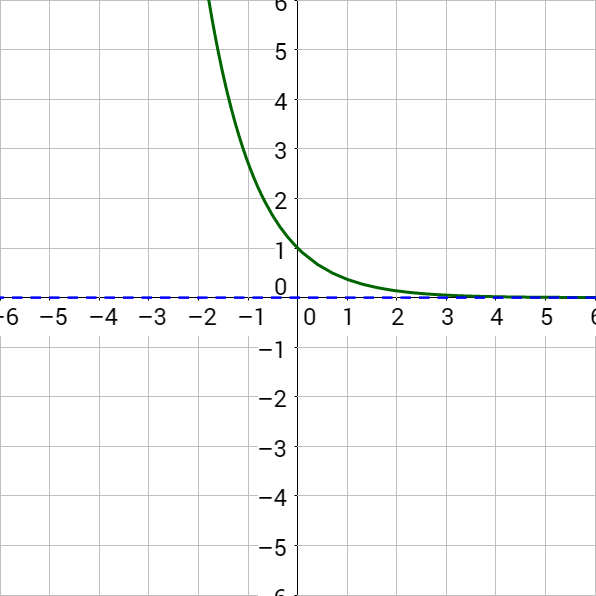;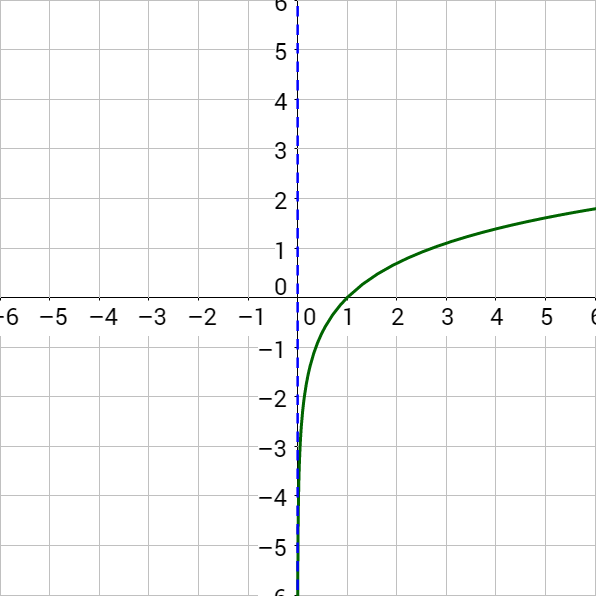;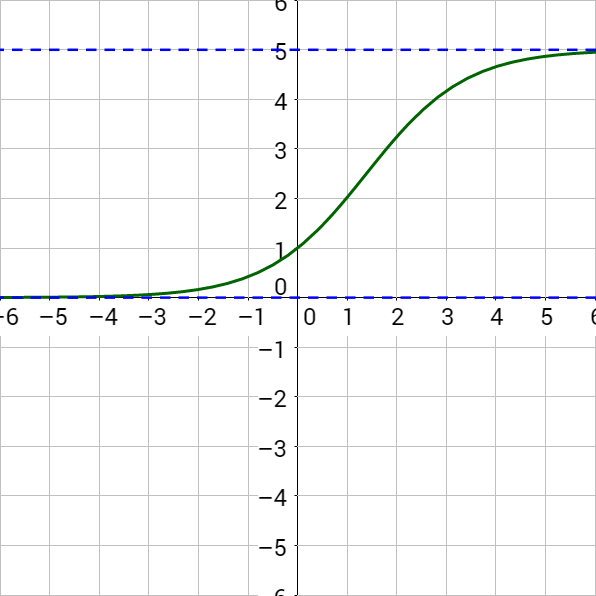;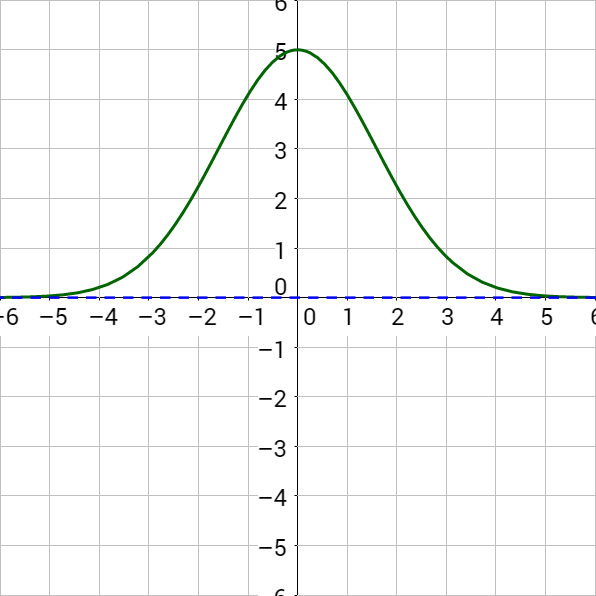3. f(t) = 4e0.231t; 1290 people
4. A = 325e−0.0462t; 1.27 mg
5. A = 250e−0.2231t; 52 apples
6. f(t) = 2250e−1.8264t; 0.039 mg; 0.759 hours
7. initial amount = 15.4 units; 200 units
8.; 7 sparrows; 45 sparrows; 8.36 years; 125 sparrows
9. 5 people; 45 people; 230 people, maximum number of people who will have heard the rumor; look it up
10.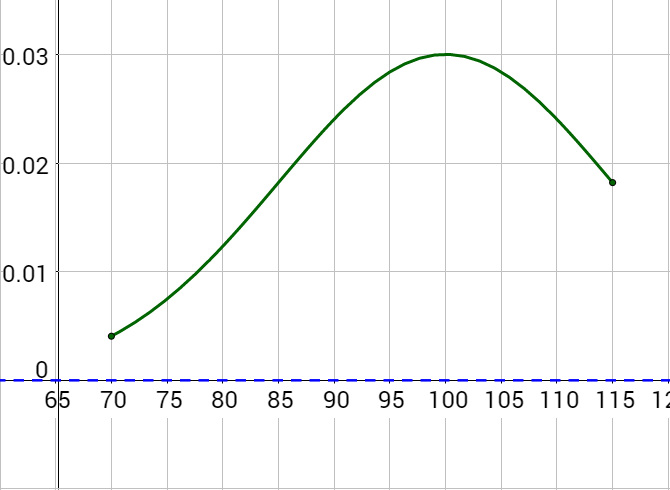; 100
11.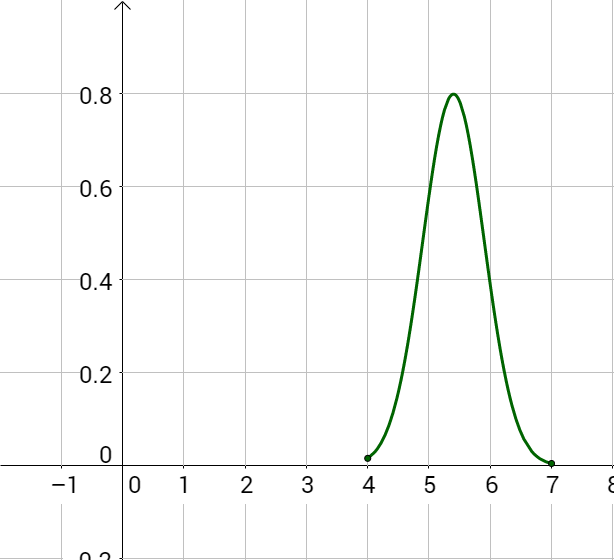; 5.4 hrs
12. 316; 630,957; 7; 4.3
13. 50 dB; 110 dB; 10−3 W/m2; 10−11 W/m2
14. logarithmic ($$y = \log_3 \left(\frac{1}{2} x\right)$$)
15. exponential (y = 1.2x)
16. x ≈ 1.080
17. t = −2.5
18. 1 + log5 r − 3 log5 t
19. x = 25 = 32
20. g(x) = −3 ⋅ 5x + 2 + 4